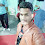# Program to get nth bit of a number

## Problem Statement:- Program to Get or Find nth Bit of a Number.

#### Sample Input/Output:-

 Sample Input First:13//Number3//Position/*13 Binary=>1101*/ Sample Output First: 1 Sample Input Second: 39//Number4//Position/*39 Binary=>100111*/ Sample Output Second: 0

#### Data requirement:-

Input Data:- number, bit_pos

Output Data:-String Output

Program in C

Code:

Program in C++

## Here is the source code of the C++ Program to Find the nth bit of a number.

Code:

### ``import java.util.Scanner;public class GetNthBit {    public static void main(String[] args) {        Scanner sc = new Scanner(System.in);        /* Get the number input */        System.out.print("Enter the Number:");        int number = sc.nextInt();        /* Get the bit position input */        System.out.print("Enter the Bit position you want to get(Between 0-31):");        int bit_pos = sc.nextInt();        /* Calculating the bit mask */        int mask = (1 << bit_pos);        /* Checking the Given Position bit is 0 or 1 */        if ((number & mask) == 1)            System.out.print("Given Position bit is 1.");        else            System.out.print("Given Position bit is 0.");        sc.close();    }}``Input/Output:Enter the Number:254Enter the Bit position you want to get(Between 0-31):1Given Position bit is 0.

Program in Python

Code:

### C/C++/java/Python practice questions with solution

1.1.